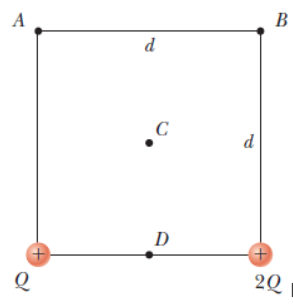# Problem: Rank the electric potentials at the four points shown in the figure below from largest to smallest. (Use only "&gt;" or "=" symbols. Do not include any parentheses around the letters or symbols.)

###### FREE Expert Solution

Electric potential:

$\overline{){\mathbf{V}}{\mathbf{=}}\frac{\mathbf{k}\mathbf{q}}{\mathbf{r}}}$

At point A, The electric potential is given as:

VA = k(Q)/d + k(2Q)/sqrt(2)d = (kQ/d)[1 + 2/sqrt(2)] = 2.414kQ/d

85% (487 ratings)###### Problem Details

Rank the electric potentials at the four points shown in the figure below from largest to smallest. (Use only ">" or "=" symbols. Do not include any parentheses around the letters or symbols.)